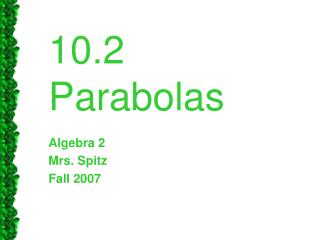DownloadDownload Presentation10.2 Parabolas

# 10.2 Parabolas

Télécharger la présentation## 10.2 Parabolas

- - - - - - - - - - - - - - - - - - - - - - - - - - - E N D - - - - - - - - - - - - - - - - - - - - - - - - - - -
##### Presentation Transcript

1. 10.2 Parabolas Algebra 2 Mrs. Spitz Fall 2007

2. Objectives • Write equations of parabolas • Graph parabolas from given information.

3. Parabolas • We already know the graph of y=ax2 is a parabola w/ vertex (0,0) and AOS x=0 • Every parabola has the property that any point on it is equidistant from a point called the Focus and a line called the directrix.

4. Focus Lies on AOS Directrix

5. The focus and directrix each lie IpI units from the vertex. (the vertex is ½ way between the focus and directrix)

6. x2=4py, p>0 Focus (0,p) Directrix y=-p

7. x2=4py, p<0 Directrix y=-p Focus (0,p)

8. y2=4px, p>0 Directrix x=-p Focus (p,0)

9. y2=4px, p<0 Directrix x=-p Focus (p,0)

10. Standard equation of Parabola (vertex @ origin)

11. Identify the focus and directrix of the parabolax = -1/6y2 • Since y is squared, AOS is horizontal • Isolate the y2→ y2 = -6x • Since 4p = -6 • p = -6/4 = -3/2 • Focus : (-3/2,0) Directrix : x=-p=3/2 • To draw: make a table of values & plot • p<0 so opens left so only choose neg values for x

12. Your Turn! • Find the focus and directrix, then graph x = 3/4y2 • y2 so AOS is Horizontal • Isolate y2→ y2 = 4/3 x • 4p = 4/3 p = 1/3 • Focus (1/3,0) Directrix x=-p=-1/3

13. Writing the equation of a parabola. • The graph shows V=(0,0) • Directrex y=-p=-2 • So substitute 2 for p

14. x2 = 4py • x2 = 4(2)y • x2 = 8y • y = 1/8 x2 and check in your calculator

15. Your turn! • Focus = (0,-3) • X2 = 4py • X2 = 4(-3)y • X2 = -12y • y=-1/12x2 to check